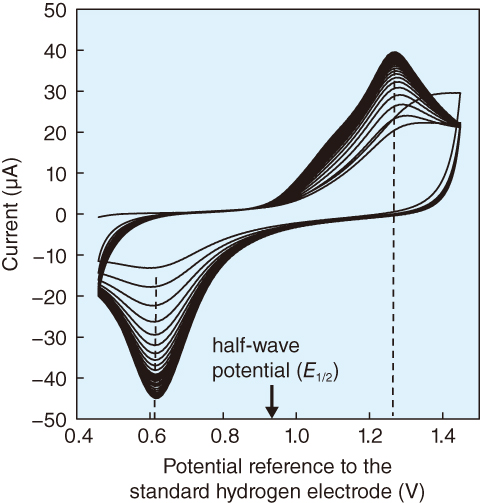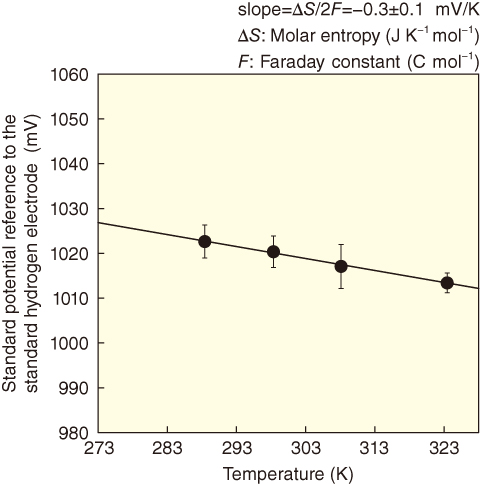# 8-10 Prediction of Radionuclide Speciation in Groundwater

## －Determination of Standard Thermodynamic Data of Se(VI)/(IV) Couple－Fig.8-25　Cyclic voltammogram of Se(VI)/(IV) couple

The growth of the redox wave indicates the increase in the active electrode area where electron transfer is possible due to the removal of the passivating layer by repetitive potential cycling. The anodic and cathodic peaks correspond to the oxidation of Se(IV) and the reduction of Se(VI), respectively.Fig.8-26　Molar entropy of Se(VI)/(IV) couple

HSeO4/H2SeO3 standard redox potential as a function of temperature. The solid line is a weighted linear regression of the data. ΔS is derived from the slope of this line.

We assess the safety of the geological disposal based on the assumption that the radionuclides contained in vitrified waste are transported to the biosphere by flowing groundwater. We obtained the necessary data for this assessment.

Selenium-79(79Se) is a long-lived fission product. Se species vary, depending on the oxidation/reduction potential E. Radionuclides are transported through the buffer material and host rocks and are retarded by sorption onto them. Because each species has unique sorption characteristics, the migration of Se depends strongly on E. Therefore, we accounted for the oxidation of the original reducing groundwater due to a decrease in the depth of repositories and/or the presence of an oxidizing agent, such as H2O2 that radiolysis of groundwater generates. The oxidation state of Se is Se(IV) or Se(VI) in oxidized groundwater. Because the sorption characteristics of Se(IV) differ from those of Se(VI), the accurate prediction of the ratio Se(VI) to Se(IV) is crucial for understanding the migration of Se. The standard redox potential E0 of the Se(VI)/(IV) couple determines Se(VI) to Se(IV) ratios at 298.15 K in calculating the Se speciation. This potential is difficult to determine because the equilibrium of the Se(VI)/(IV) couple, whose reaction rate is slow, is not rapidly established, and the experimentally determined E is not affected by the Se(VI) to Se(IV) ratio in solution. We therefore chose to use cyclic voltammetry CV, which is a common method used to measure the half-wave potential E1/2. The potential E1/2 is where the concentration of an oxidant equals that of a reductant. Even for the redox reaction with a slow rate, CV allows us to measure E1/2. Fig.8-25 shows the cyclic voltammogram of the Se(VI)/(IV) couple, where E1/2 is midway between the anodic and cathodic peak potentials. The value of E0 is derived from the concentration dependence of E1/2.

In the geological environment, temperatures exceed 298 K. Because the molar entropy of the reaction ΔS yields the temperature derivatives of E0, ΔS of the Se(VI)/(IV) couple is also required to predict the Se(VI) to Se(IV) ratio at a temperature above 298 K. We therefore obtained ΔS for this couple from the temperature dependence of E0 (Fig.8-26). The results for E0 and ΔS, which agree with publised values of the molar enthalpy of the Se(VI)/(IV) couple, will contribute to a more reliable prediction of Se speciation under various environments and will be input in the JAEA thermodynamic database.

| | | | |